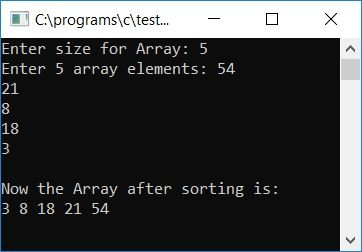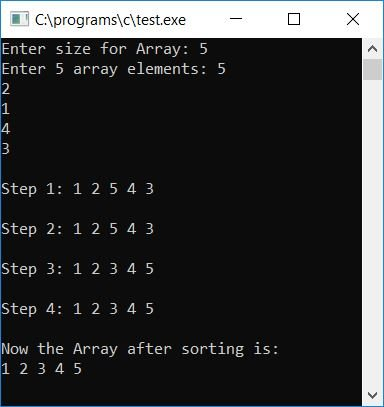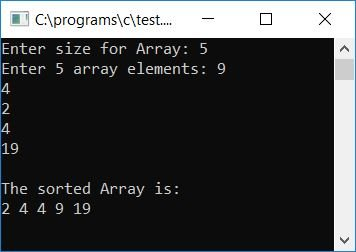# C Program for Selection Sort

In this tutorial, we will learn how to create a program in C that sorts an array using the selection sort technique. At last, we have also created a function that can be used to sort any given array in ascending order using the selection sort technique.

But before going through the program, if you are not aware of how selection sort works, refer to the step-by-step workings of selection sort. Now let's move on and implement it in a C program.

## Selection sort in C

To sort an array in ascending order using the selection sort technique in C programming, you have to ask the user to enter the array's size and its elements. Then apply the selection sort mechanism and print the sorted array as output, just like the program given below:

```#include<stdio.h>
#include<conio.h>
int main()
{
int size, arr, i, j, temp, small, count=0, index;
printf("Enter size for Array: ");
scanf("%d", &size);
printf("Enter %d array elements: ", size);
for(i=0; i<size; i++)
scanf("%d", &arr[i]);
for(i=0; i<(size-1); i++)
{
small = arr[i];
for(j=(i+1); j<size; j++)
{
if(small>arr[j])
{
small = arr[j];
count++;
index = j;
}
}
if(count!=0)
{
temp = arr[i];
arr[i] = small;
arr[index] = temp;
}
count=0;
}
printf("\nNow the Array after sorting is:\n");
for(i=0; i<size; i++)
printf("%d ", arr[i]);
getch();
return 0;
}```

As the above program was written in the Code::Blocks IDE, here is the sample run after a successful build and run. This is the first snapshot of the sample run:Now enter the array size, say 5, and its elements as 54, 21, 8, 18, and 3, and press the ENTER key to see the sorted array shown in the second snapshot of the sample run provided here:Here is another sample run. This is the final snapshot:## C Selection Sort Program Explained

• At runtime, get the array size from the user.
• Then receive elements of that size for the array at run-time.
• For example, if the user has supplied 5 as the array size, then ask him or her to enter 5 array elements.
• Create a for loop that runs from 0 to one less than the size of the array. That is, if the array's size is 5, then run the loop from 0 to one less than 5-1  or 4.
• Set the element at the current index to a variable, let's call it "small," inside the loop.
• And using another for loop (which runs from 0 to one less than the size of the array), check whether the value in the small variable is greater than any element (present at index equals to the value of i+1 to size-1 or 4) or not.
• If it is, set that element to a "small" variable and increase the "count" variable. Then, set the current value of j (the inner loop variable) to the "index" variable, which holds the index number where the smallest element appears.
• Never forget to initialize the "count" (with 0) variable at the start of the program.
• Now, after exiting from the inner for loop, check whether count holds any value other than 0 or not.
• If it holds any value other than 0, then program flow has gone inside the if statement of the inner for loop. And it means that any element that was initialized to the "small" variable.
• Therefore, we have to perform a swapping operation, that is, place the element at the beginning of any variable, say "temp,"  and then initialize the value of "small" at the beginning. At the end, the value of "temp" is initialized at the index from where the element was initialized to the "small" variable before swapping.
• After swapping, initialize 0 to the "count" variable and continue.
• For example, if the user has entered 5 as the array size and "54 21 8 18 3" as its elements.
• Then, at the first run of the "for" loop, "i" holds 0. Furthermore, "i<(size-1)" or "0<0(5-1)" or "0<4" evaluates to true. Therefore, program flow goes inside the loop.
• The value at "arr" or "54" gets initialized to "small." Therefore, "small" holds 54
• Now, in the inner "for" loop, the value of "i+1" (0+1) or "1" gets initialized to "j" (the inner loop's variable). Therefore, "j" holds 1, and 1 is less than "size" or "5". Therefore, program flow goes inside the inner "for" loop.
• Now the statement "small>arr[j]" or "54>arr" or "54>21" is evaluated to be true, therefore "arr[j]" or "arr" or "21" gets initialized to "small". The value of "count" gets incremented and becomes 1, and the value of "j" or "1" gets initialized to "index."
• Again the value of "j" gets incremented and compared to see whether "j" is less than "size" or not, that is "j (2)" is less than "size(5)", therefore again program flow goes inside the loop.
• And again, "small>arr[j]" or "21>arr" or "21>8" evaluates to true, therefore, after swapping
• The variable "small" holds "8," "count" holds "2," "index" holds "2."
• Again the value of "j" gets incremented and compared to see whether "j" is less than "size" or not, that is "j (3)" is less than "size(5)," therefore again program flow goes inside the loop.
• And again, "small>arr[j]" or "8>arr" or "8>18" evaluates to false, therefore, no swapping gets performed.
• The variable "small" holds "8," "count" holds "2," "index" holds "2."
• Again the value of "j" gets incremented and compared to see whether "j" is less than "size" or not, that is "j (4)" is less than "size(5)", therefore again program flow goes inside the loop.
• And again, "small>arr[j]" or "8>arr" or "8>3" evaluates to true, therefore, after swapping
• The variable "small" holds "3," "count" holds "3," "index" holds "4."
• Again value of "j" gets incremented and compared to determine whether "j" is less than "size" or not, that is, whether "j (5)" is less than "size(5)" or not. Here the condition evaluates to false, and the program flow goes back at the outer "for" loop and increments the value of "i." Then checks the condition to see whether "i" is less than "size-1," "5-1," or "4" or not. It evaluates to true, so the program flow returns to the loop and repeats the preceding steps until the condition of the outer "for" loop is evaluated as false.

If you want to print array elements as a step-by-step sorting of array elements shown on the output screen along with its final array, Then you can modify the above code as shown below to print array elements after each sorting step:

```#include<stdio.h>
#include<conio.h>
int main()
{
int size, arr, i, j, temp, small, count=0, index;
printf("Enter size for Array: ");
scanf("%d", &size);
printf("Enter %d array elements: ", size);
for(i=0; i<size; i++)
scanf("%d", &arr[i]);
for(i=0; i<(size-1); i++)
{
small = arr[i];
for(j=(i+1); j<size; j++)
{
if(small>arr[j])
{
small = arr[j];
count++;
index = j;
}
}
if(count!=0)
{
temp = arr[i];
arr[i] = small;
arr[index] = temp;
}
printf("\nStep %d: ", i+1);
for(j=0; j<size; j++)
printf("%d ", arr[j]);
printf("\n");
count=0;
}
printf("\nNow the Array after sorting is:\n");
for(i=0; i<size; i++)
printf("%d ", arr[i]);
getch();
return 0;
}```

Here is the final snapshot of the sample run:### C selection sort using a user-defined function

Let's create the same program, which is to sort an array in ascending order using the selection sort technique, but this time with the help of a function. Here selection sort is implemented inside a function named selsort() that uses two arguments, one of which is an array and the other is its size, as shown in the program given below:

```#include<stdio.h>
#include<conio.h>
void selsort(int arr[], int size);
int main()
{
int size, arr, i;
printf("Enter size for Array: ");
scanf("%d", &size);
printf("Enter %d array elements: ", size);
for(i=0; i<size; i++)
scanf("%d", &arr[i]);
selsort(arr, size);
printf("\nThe sorted Array is:\n");
for(i=0; i<size; i++)
printf("%d ", arr[i]);
getch();
return 0;
}
void selsort(int arr[], int size)
{
int i, j, temp, small, count=0, index;
for(i=0; i<(size-1); i++)
{
small = arr[i];
for(j=(i+1); j<size; j++)
{
if(small>arr[j])
{
small = arr[j];
count++;
index = j;
}
}
if(count!=0)
{
temp = arr[i];
arr[i] = small;
arr[index] = temp;
}
count=0;
}
}```

Here is the final snapshot of the above program:The concept used in the above program is similar to that used in the first program, except we have implemented the function selsort() here. This function implements all selection sorting techniques. We only have to pass any array and its size as an argument to this function. And all the elements inside the array get sorted in ascending order.

#### The same program in different languages

C Quiz

« Previous Program Next Program »